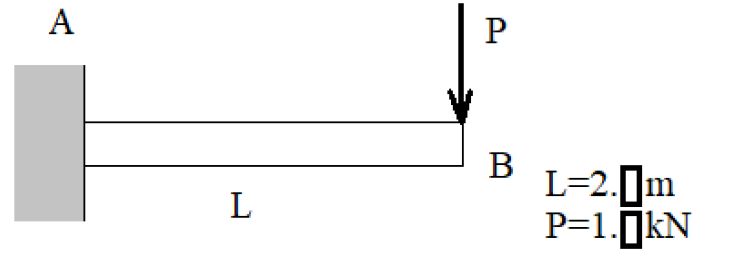# Question The anchor beam AB is of uniform cross-section and carries a load P at the free end B. Determine the equation of the elastic curve and the displacement and slope at B. (2.8 m 1.8 kn) A P B L L=2.Om P=1.OkNTLOP1P The Asker · Other Mathematics

The anchor beam AB is of uniform cross-section and carries a load P at the free end B. Determine the equation of the elastic curve and the displacement and slope at B. (2.8 m 1.8 kn)Transcribed Image Text: A P B L L=2.Om P=1.OkN
More
Transcribed Image Text: A P B L L=2.Om P=1.OkN